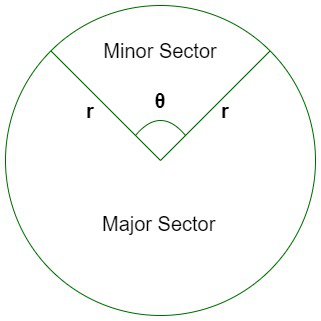GeeksforGeeks App
Open AppBrowser
Continue

# How to calculate the Area of a Sector of Circle?

Sector is defined as the portion inside a circle bounded between two radii and the arc of the circle. The area of this sector depends upon the corresponding angle between the two radii.

Properties:

• A sector of a circle always originates from the center of the circle.
• The semi-circle is the most common sector of a circle, with the angle between the radii equal to 180°.
• The area of the semi-circle depends upon the radii of the circle.

Types of Sectors

There are three types of sectors depending upon the angle between the corresponding two radii of the sector. They are:

• Minor sector: When the angle enclosed between the two radii is less than 180°. The area enclosed is also smaller than a semi-circle.
• Semi-circle: When the angle enclosed between the two radii is equal to 180°.
• Major sector: When the angle enclosed between the two radii is greater than 180°. The area enclosed is also greater than a semi-circle.

Formula for Area of a Sector

For a circle having radius equals to ‘r’ units and angle of the sector is θ (in degrees), the area is given by,Area of sector = θ / 360° × πr2

When θ is given in radian, the area is given by

Area of sector = 1/2 × r2θ

Proof:

For a circle with radius r units, the area is given by πr2.

Now the fraction of the area enclosed by the sector will be the same as the fraction of the angle enclosed by the sector in the circle.

Thus, the fraction of area enclosed = θ / 360°

So, the area enclosed by the sector = (θ / 360°) × πr2

### Sample Problems

Question 1. Find the area of the sector of a circle whose angle enclosed equals 60o and the radius of the circle is 5 units. It is a major or minor sector?

Solution:

Give, the angle of the sector = θ = 60°

The radius of the circle = 5 units

Thus, the area of the sector = 60°/360° × π × 52 = 25π/6

Approximating the value of π = 3.14, we get,

Area of sector = 25 × 3.14 / 6 = 13.08 sq. units

Since, angle of sector is less than 180°, it is a minor sector.

Question 2. Find the area of a sector whose angle is given as π/2 radians and the radii of the circle is 8cm.

Solution:

Since the angle of the sector is given in radian, we can write,

Area of the sector = 1/2 × r2 × θ

Given, the radius of the circle is 8cm. Thus,

Area of sector = 1/2 × 82 × π/2 = 16π cm2

Approximating the value of π = 3.14, we get,

Area of sector = 16 × 3.14 = 50.24 cm2

Question 3. For a circle of a given area 50cm2, there are three sectors of area 25cm2, 45cm2, and 13cm2. Classify the given sectors among the minor sector, semi-circle, and major sector.

Solution:

The area of the circle is 50cm2.

Thus, half of the area of the circle is 50/2 = 25cm2

Thus, the sector with an area of 25cm2 is a semi-circle.

The sector with an area of 45cm2 has a greater area than a semi-circle. Thus, it is a major sector.

Lastly, the sector with an area of 13cm2 has a smaller area than a semi-circle. Thus, it is a minor sector.

Question 4. If a pizza of radius 5 inches is divided into 6 equal slices, find the area enclosed and angle of each slice of pizza.

Solution:

Since we divide a pizza into 6 equal pieces, each piece represents a sector with an angle equal to one-sixth of the total angle of pizza, that is 360o.

So, the angle of each pizza slice = 360°/6 = 60°.

So, the area of each sector is given by,

Area of each slice = (θ / 360°) × πr2,

where,

θ = 60°

r = 5 inches

Thus, we get, area of each slice = 60°/360° × π × 52 = 25π/6 sq. inch

Putting the value of π = 3.14, we get

Area of each slice =  25 × 3.14 / 6 = 13.08 sq. inch

My Personal Notes arrow_drop_up
Related Tutorials